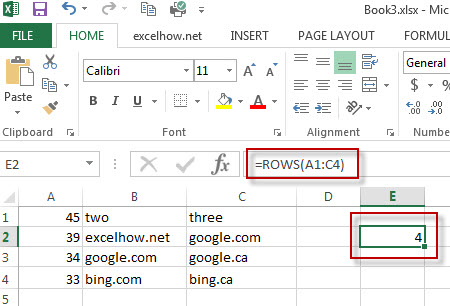# Excel Rows Function

This post will guide you how to use Excel ROWS function with syntax and examples in Microsoft excel.

## Description

The Excel ROWS function returns the number of rows in a cell reference.

The ROWS function is a build-in function in Microsoft Excel and it is categorized as a Lookup and Reference Function.

The ROWS function is available in Excel 2016, Excel 2013, Excel 2010, Excel 2007, Excel 2003, Excel XP, Excel 2000, Excel 2011 for Mac.

## Syntax

The syntax of the ROWS function is as below:

`= ROWS(array)`

Where the ROWS function arguments is:
array-This is an required argument. An array, or A reference to a range of cells.

## Example

The below examples will show you how to use Excel ROWS Lookup and Reference Function to return the number of rows in a range of cells.

#1 To get the number of rows in the cell range “A1:C4”, just using the following excel formula: =ROWS(A1:C4)### More Excel Rows Examples

• Flip a Column of Data Vertically
How do I remove time part from a date in Excel. How to get only date part from the date with time values via Format cells feature or Excel function. How to extract date from a date and time with VBA code in Excel….
• Randomly Select Cells
If you want to randomly select cells from a range of cells, you can use a formula based on the INDEX function, the RANDBETWEEN function and the Rows function…..
• List all Worksheet Names
Assuming that you have a workbook that has hundreds of worksheets and you want to get a list of all the worksheet names in the current workbook. And the below will introduce 3 methods with you..…
• Split Data in One Column to Multiple Columns
If you want to split each sets in one column from row to row in stacked columns, and you need to create a formula based on the OFFSET function, the COLUMNS function, and the ROWS function..…
• Copy and Paste Only Non-blank Cells
If you want only copy non-blank cells in a range in Excel, you need to select the non-blank cells firstly, then press Ctrl +C keys to copy the selected cells. So how to only select all non-blank cells in the selected range in your worksheet..…